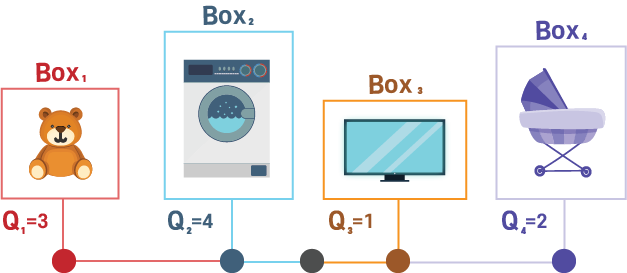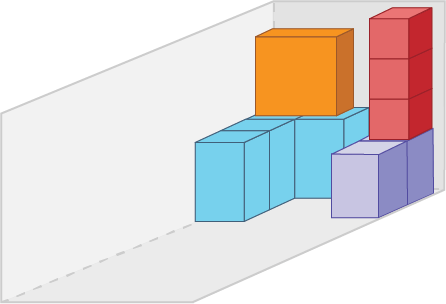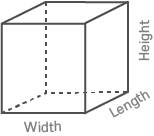## Save time and avoid mistakes

### Are you tired of calculating your clients stuffing plans?VΣ = 3.21 м3

Make it easy, fast and get the best cargo load planning optimization to provide the cheapest shipping costs and win every deal

Example$V = L × V × H$
Q1 = 3 boxes
Q3 = 1 box
Q2 = 4 boxes
Q4 = 2 boxes

B1:

$L$1 = 400 мм
$W$1 = 400 мм
$H$1 = 420 мм
$V$1 = 0.07 м3
$V$1 Σ = V1 × Q1
$V$1 Σ = 0.21 м3

B2:

$L$2 = 650 мм
$W$2 = 680 мм
$H$2 = 1200 мм
$V$2 = 0.53 м3
$V$2 Σ = V2 × Q2
$V$2 Σ = 2.12 м3

B3:

$L$3 = 1400 мм
$W$3 = 300 мм
$H$3 = 1000 мм
$V$3 = 0.42 м3
$V$3 Σ = V3 × Q3
$V$3 Σ = 0.42 м3

B4:

$L$4 = 800 мм
$W$4 = 550 мм
$H$4 = 520 мм
$V$4 = 0.23 м3
$V$4 Σ = V4 × Q4
$V$4 Σ = 0.46 м3
$V$Σ = V1 + V2 + V3 + V4
$V$Σ = 0.46 + 0.42 + 2.12 + 0.21
$V$Σ = 3.21 м3

Still waiting rates
from Freight Forwarders...